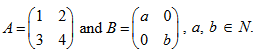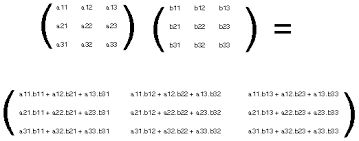## Filters

Sort by :
Clear All
Q
Engineering
118 Views   |

LetThen

• Option 1)

there cannot exist any B such that AB = BA

• Option 2)

there exist more than one but finite number B's such that AB = BA

• Option 3)

there exists exactly one B such that AB = BA

• Option 4)

there exist infinitely many B's such that AB = BA

As we learnt in

Multiplication of matrices --

2b = 2a

3a  = 3b

Option 1)

there cannot exist any B such that AB = BA

Incorrect Option

Option 2)

there exist more than one but finite number B's such that AB = BA

Incorrect Option

Option 3)

there exists exactly one B such that AB = BA

Incorrect Option

Option 4)

there exist infinitely many B's such that AB = BA

Correct Option

View More
Engineering
120 Views   |

If A and B are square matrices of size n x n such that then which of the following will be always true?

• Option 1)

A = B

• Option 2)

AB = BA

• Option 3)

either A or B is a zero matrix

• Option 4)

either A or B is an identity matrix

As we learn tin

Multiplication of matrices --

Option 1)

A = B

Incorrect option

Option 2)

AB = BA

Correct option

Option 3)

either A or B is a zero matrix

Incorrect option

Option 4)

either A or B is an identity matrix

Incorrect option

View More
Exams
Articles
Questions